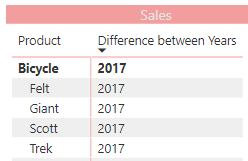I had a requirement where the customer wanted the difference between 2 values in a table.

The challenge here was that because the values are already part of the table, I had to find a way to get the unique value for each item. This would then allow me to calculate each value separately and then calculate the difference.

This is what the final output looked like, and below is the DAX measure that I created to get the difference.

As my good friend Matt always says break it down into smaller parts to solve the puzzle. In this instance I know that I needed to have two measures, where one would be filtered for the first year and the second one would be filtered to the last year.

```Difference between Years =
VAR AllYears =
CALCULATE (
CONCATENATEX (
VALUES ( 'Table1'[Year] ),
'Table1'[Year] ,
","
)
)
VAR FirstYear =
RIGHT ( AllYears, 4 )
VAR LastYear =
LEFT ( AllYears, 4 )
VAR FirstYearAmount =
CALCULATE (
[Sales],
'Table1'[Year] =  FirstYear
)
VAR LastYearAmount =
CALCULATE (
[Sales],
'Table1'[Year] = LastYear
)
RETURN    FirstYearAmount – LastYearAmount
```

Here is the explanation of my measure

• Lines 2 – 9
• I am getting a concatenated list of all the years that are selected separated by the comma
• EG: For 2017 and 2018 it is returning the following.
•• Lines 10 – 11
• This is where I am getting the first year from my concatenated list of years
•• Lines 12 – 13
• This is where I am getting the last year from my concatenated list of years
•• Lines 14 – 18
• This is where I am creating my measure which will be for the first-year amount
•• Lines 19 – 23
• This is where I am creating my measure which will be for the last-year amount
•• Lines 24
• This allowed me now to subtract one measure from the other.
• And return the final result
•This will currently work, if multiple years are selected it will always select the first and last year from the entire selection.

As always, I hope that you enjoyed this blog, and if you got another way to get the same result I would be interested in hearing about it in the comments below.